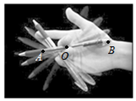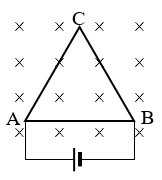## 徐汇区2022学年第一学期期末

•  2023/3/2
•  146 次
•  2881 K

1．下列物理量在国际单位制中，属于基本物理量的是

（A）电场强度              （B）磁感强度              （C）电流强度              （D）电荷量

【答案】

C

2．某原子核 X 释放出一个电子后转变为新核 Y，可知衰变前后核内

（A）电子数减少 1     （B）质子数减少 1      （C）中子数减少 1        （D）质量数减少 1

【答案】

C

3．一肥皂膜竖直放置在单色点光源 S 与光屏之间，如图所示，光屏上的圆形区域内应

（A）比周围稍亮，且明暗均匀         （B）比周围稍亮，且明暗相间

（C）比周围稍暗，且明暗均匀         （D）比周围稍暗，且明暗相间

【答案】

D？C?

4．一平面简谐横波以 v = 1 m/s 的速度沿 x 轴正方向传播，t = 0 时刻的波形图如图所示。则 t = 2 s 时，质点 A 将做

（A）加速度增大的减速运动              （B）加速度减小的减速运动

（C）加速度增大的加速运动              （D）加速度减小的加速运动

【答案】

A

5．如图，a、b 两点位于以真空中一静止的负点电荷为球心的球面上，c 点在球面外，则

（A）a 点 b 点场强相等            （B）b 点场强比 c 点小

（C）a 点电势比 b 点高            （D）b 点电势比 c 点低

【答案】

D

6．一只可视为质点的蜜蜂沿弯曲轨迹做匀速率运动，蜜蜂在途经 A、B、C、D 位置时的速度 v 和所受合力 F 的大小、方向如图所示，其中可能正确的是

（A）A 或 C          （B）B 或 D

（C）A 和 C          （D）B 和 D

【答案】

B

7．同型号的废旧玩具直流电动机 a、b、c、d，内部结构如图。某同学先后将各台电动机的正负接线柱用导线直接相连，并用力扭转它们的转轴，发现 a 转得越快越省力，b 转得越快越费力，c 转动快慢阻力都一样，d 无法转动，可知其中仍能正常工作的是

（A）a            （B）b            （C）c            （D）d

【答案】

B

8．某转笔高手能让笔绕其上的某一点 O 匀速转动，笔杆上相距为 L 的 A、B 两点，在如图时刻的速度大小分别为 vAvB，向心加速度大小为 aAaB，OB 两点间为距离 rB，则有

（A）$$\frac{{{a_{\rm{A}}}}}{{{a_{\rm{B}}}}}$$ = $$\frac{{{v_{\rm{A}}}}}{{{v_{\rm{B}}}}}$$，rB = $$\frac{{{v_{\rm{A}}}L}}{{{v_{\rm{A}}} + {v_{\rm{B}}}}}$$         （B）$$\frac{{{a_{\rm{A}}}}}{{{a_{\rm{B}}}}}$$ = $$\frac{{v_{\rm{A}}^2}}{{v_{\rm{B}}^2}}$$，rB = $$\frac{{{v_{\rm{A}}}L}}{{{v_{\rm{A}}} + {v_{\rm{B}}}}}$$

（C）$$\frac{{{a_{\rm{A}}}}}{{{a_{\rm{B}}}}}$$ = $$\frac{{{v_{\rm{A}}}}}{{{v_{\rm{B}}}}}$$，rB = $$\frac{{{v_{\rm{B}}}L}}{{{v_{\rm{A}}} + {v_{\rm{B}}}}}$$          （D）$$\frac{{{a_{\rm{A}}}}}{{{a_{\rm{B}}}}}$$ = $$\frac{{v_{\rm{A}}^2}}{{v_{\rm{B}}^2}}$$，rB = $$\frac{{{v_{\rm{B}}}L}}{{{v_{\rm{A}}} + {v_{\rm{B}}}}}$$

【答案】

C

9．某同学用手机内部的磁传感器测量地磁场磁感强度大小 B0，测得如图手机坐标系中 xyz 三个方向上的磁感强度分别为 Bx = 0，By = 20 μT，Bz = − 46 μT，若将手机边框看作闭合线圈，则

（A）B0 ≈ 66 μT；绕 x 轴旋转手机时边框中无感应电流

（B）B0 ≈ 66 μT；绕 z 轴旋转手机时边框中无感应电流

（C）B0 ≈ 50 μT；绕 x 轴旋转手机时边框中无感应电流

（D）B0 ≈ 50 μT；绕 z 轴旋转手机时边框中无感应电流

【答案】

D

10．三条相同的电阻丝组成等边三角形导体框 ABC 垂直磁场放置，接入如图电路后线框受到的安培力的合力大小为 F，其中 AC 边受到的安培力大小为

（A）F/4                （B）F/3                （C）F/2                （D）2F/3

【答案】

B

11．如图所示，一根均匀带电的长直橡胶棒沿其轴线方向做速度为 v 的匀速直线运动。已知棒的横截面积为 S，单位体积所带的电荷量为 − q。由于棒的运动而形成的等效电流

（A）大小为 qSv，方向与 v 相反            （B）大小为 qSv，方向与 v 相同

（C）大小为 qv，方向与 v 相反              （D）大小为 qv，方向与 v 相同

【答案】

A

12．下列图像中，可表示地面附近一小球沿竖直方向做抛体运动过程中，速度 v 与重力势能 Ep 之间关系的是（不计空气阻力）【答案】

A

13．由大量氘（21H）核组成的高温等离子体中，可以发生两个氘核聚合为一个新核并放出一个中子的核反应，该反应的方程式为__________________________；为发生这一反应，每个核至少要有 72 keV 的动能才能克服库仑斥力靠近并发生聚合，同一时刻等离子体中有多少比例的氘核满足这一反应条件，在宏观上对应的物理量是_______。

【答案】

221H → 32He + 10n；等离子体的温度

14．在光刻机内部，芯片电路的紫外线影像通过一系列复杂的光路和狭小的孔径，微缩到几纳米的尺度后在硅晶片表面曝光蚀刻出实际电路。紫外线波长越短，衍射越________，为得到更小的曝光尺寸就需要波长更短的紫外线，对光刻机可采取的改进措施为____________。

【答案】

15．物体在星球表面附近绕星球做匀速圆周运动的速度叫做该星球的第一宇宙速度，对月球可近似认为其第一宇宙速度 v1 = $$\sqrt {gR}$$，其中 R 表示月球半径，g 表示_______________。不计其它天体的引力作用，若在月球表面以 v1 为初速度竖直向上抛出一石块，该石块所能上升的最大高度 H________R/2（选填“>”、“<”或“=”）。

【答案】

16．一带电小球只在重力、电场力和空气阻力作用下从 A 到 B 运动。运动过程中小球的机械能 E、重力势能 Ep、动能 Ek、电势能 E、重力做功 WG、电场力做功 W、空气阻力做功 Wf 中的部分物理量大小或变化情况已在下表中列出，试完成该表：

 E Ep Ek ΔE电 WG W电 Wf A 5.5 J − 3 J 8.5 J 2 J B 7 J 2 J

【答案】

5 J，− 2 J，− 5 J，− 0.5 J

17．某同学尝试“吸”开“马德堡半球”。如图所示，一内壁光滑的导热气缸固定在水平地面上，一横截面积 SA、质量 M 的活塞将一定质量的理想气体和一个圆柱形“马德堡半球”装置密封在气缸内。圆柱形“马德堡半球”竖直悬挂，“半球”内部已近似抽成真空，上下两片“半球”质量均为 m，中空部分横截面积为 SB，忽略两半球间的接触面积及壁厚，两片合并后所占据的总体积为 VB。初始时，缸内气体压强等于大气压强 p0，气缸与活塞之间的容积为 VA，重力加速度为 g。用水平向右的拉力缓慢拉动活塞，当拉力大小 F =________时，两“半球”恰自动分开；在此过程中活塞向右移动的距离 d =________。

【答案】

p0SA − $$\frac{{mg{S_{\rm{A}}}}}{{{S_{\rm{B}}}}}$$；$$\frac{{({p_0}{S_{\rm{B}}} - mg)({V_{\rm{A}}} - {V_{\rm{B}}})}}{{mg{S_{\rm{A}}}}}$$

18．在“测量电源电动势和内电阻”的实验中，可供选择的实验器材（均可视为理想电表或理想元件）有：电压表（量程 0 ~ 3 V，最小刻度 0.1 V）、电流表（量程 0 ~ 0.6 A，最小刻度 0.02 A）、滑动变阻器（最大阻值 30 Ω）、电阻箱（调节范围 0 ~ 9999 Ω）、电键及导线若干。

（1）某组同学选取部分元件组成如图（a）所示电路，并在 U I 图中描绘测得的数据点如（b）图所示 5 组数据。可知待测电源电动势约为_______V，内电阻约为_______Ω；实验过程中滑动变阻器接入电路的最大阻值约为_______Ω，滑动变阻器的最大功率约为________W；

（2）另一组同学计划测量一“可乐电池”（电动势小于 1 V，内电阻约为几 kΩ）的电动势和内电阻，应从上述器材中选取__________________。不选择其余器材的理由是：

______________________________________________________________________________。

【答案】

（1）1.4；0.8；27.2；0.46

（2）电压表、电阻箱、电键及导线若干；

19．如图，与水平面夹角 θ = 37° 的斜面与半径 R = 0.4 m 的光滑圆轨道的最低点 B 圆滑对接，且固定于竖直平面内。小滑块从斜面上的 A 点由静止释放，经 B 点后沿圆轨道运动，通过最高点 C 时轨道对滑块的弹力恰为零。已知滑块与斜面间动摩擦因数 μ = 0.25。g 取 10 m/s2，sin37° = 0.6，cos37° = 0.8。求：

（1）滑块在 C 点的速度大小 vC

（2）A、B 两点间的高度差 h

（3）滑块在 BC 段的运动过程中，是否存在动能和势能相等的位置？若有，对零势能面的选取有何要求？

【答案】

（1）vC = 2 m/s

（2）h = 1.5 m

（3）i）若取 B 点上方 0.6 m 到 B 点下方 1 m 范围内任意高度为零势能面，在 BC 间存在某位置滑块动能与势能相等；

ii）若取 B 点上方 0.6 m 以上或 B 点下方 1 m 以下某高度为零势能面，在 BC 间不存在滑块动能与势能相等的位置。

20．某电动汽车在刹车过程中，驱动电机在一定条件下会处于发电状态，将车辆的部分动能转化为电能为车载电池充电；同时，为保持刹车的平顺性，除电机的电磁制动外，车辆的液压摩擦制动装置也给车辆提供一部分制动力，该过程的工作原理可简化如图：

（1）为使充电模块工作，导体棒减速滑行的初速度 v0 至少应为多大？

（2）求导体棒以上述 v0 运动时，流过导体棒的电流 I 的大小方向；（3）求导体棒从上述 v0 减速到零的过程中，液压摩擦制动力 F 随时间 t 变化的关系，并画出相应的 F t 图；

（4）简述导体棒从上述 v0 减速到零的过程中，导体棒上做功与能量转化的关系。

【答案】

（1）v0 ≥ $$\frac{{{U_s}}}{{Bl}}$$

（2）I = $$\frac{P}{{Bl{v_0}}}$$，方向由 b 向 a

（3）F = ma − $$\frac{P}{{{v_0} - at}}$$（0 ≤ t ≤ $$\frac{{{v_0}}}{a}$$  $$\frac{{{U_s}}}{{Bla}}$$）

F = ma （$$\frac{{{v_0}}}{a}$$  $$\frac{{{U_s}}}{{Bla}}$$ < t ≤ $$\frac{{{v_0}}}{a}$$）（4）导体棒的动能（或机械能）共减少 $$\frac{1}{2}$$mv02，其中 P($$\frac{{{v_0}}}{a}$$  $$\frac{{{U_s}}}{{Bla}}$$) 通过克服安培力 FA 做功转化为电能，剩余 $$\frac{1}{2}$$mv02 P($$\frac{{{v_0}}}{a}$$  $$\frac{{{U_s}}}{{Bla}}$$) 通过克服液压摩擦制动力 F 做功转化为内能。

2006 - 2023，推荐分辨率1024*768以上，推荐浏览器Chrome、Edge等现代浏览器，截止2021年12月5日的访问次数：1872万9823 站长邮箱